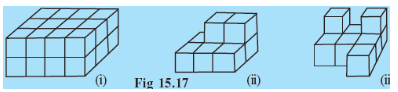# Try to guess the number of cubes in the following arrangements (Fig 15.17).i) There are 4 columns and 2 rows in the first line.
So, the total  number of cubes in the first line isAnd there is total 3 such line.
Therefore, the total number of cubes areii) There are 3 columns and 1 row in the first line at the bottom.
So, the total number of cubes in the first line areAnd there are total 2 such lines
So, the total number of cubes at the bottom areNow, we can see that two cubes are placed on the second line
Therefore, the total number of cubes areiii)   There are 3 columns and 1 row in the first line at the bottom.
So, the total number of cubes in the first line areAnd there are total 2 such lines
So, the total number of cubes at the bottom areNow, we can see that two cubes are placed on the second line  and one cube in the front of the first line
Therefore, the total number of cubes are## Related Chapters

### Preparation Products

##### Knockout JEE Main April 2021

An exhaustive E-learning program for the complete preparation of JEE Main..

₹ 22999/- ₹ 14999/-
##### Knockout NEET May 2021

An exhaustive E-learning program for the complete preparation of NEET..

₹ 22999/- ₹ 14999/-
##### Knockout JEE Main April 2022

An exhaustive E-learning program for the complete preparation of JEE Main..

₹ 34999/- ₹ 24999/-
##### Test Series NEET May 2021

Take chapter-wise, subject-wise and Complete syllabus mock tests and get in depth analysis of your test..

₹ 6999/- ₹ 4999/-